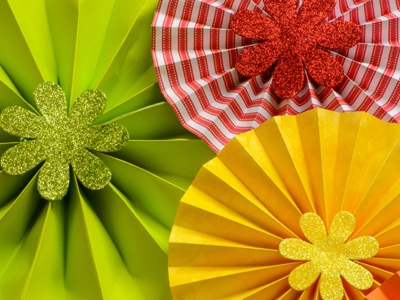The circumference of a circle is the distance all round the edge of it.

# Words Used in Math

This English Language quiz is called 'Words Used in Math' and it has been written by teachers to help you if you are studying the subject at middle school. Playing educational quizzes is a fabulous way to learn if you are in the 6th, 7th or 8th grade - aged 11 to 14.

It costs only \$12.50 per month to play this quiz and over 3,500 others that help you with your school work. You can subscribe on the page at Join Us

Perimeter and prime number are words predominantly used in Math. Math uses various English words that are rarely used elsewhere - in fact Math almost has a language all of its own!

Have a go at this quiz to get you up to speed with mathematical terms and spelling.

1.
Choose the correct meaning for the following word(s).
Perpendicular
At a reflex angle to a line
At a right angle to a line
At an acute angle to a line
At an obtuse angle to a line
Make sure you can spell 'perpendicular' correctly
2.
Choose the correct meaning for the following word(s).
Prime number
Number divisible only by itself and one
Number divisible only by itself and two
Number divisible only by itself and three
Number divisible only by itself and four
Some examples of prime numbers are 3, 5, 7, 11, 13
3.
Choose the correct meaning for the following word(s).
Integer
One letter
One whole number
The whole
The whole range of letters
'Integer' means 'whole number' (not a fraction)
4.
Choose the correct meaning for the following word(s).
Product
Result of adding two or more numbers
Result of dividing two or more numbers
Result of multiplying two or more numbers
Result of subtracting two or more numbers
The product of 2 x 2 is 4
5.
Choose the correct meaning for the following word(s).
Circumference
Distance all round the edge of a circle
Distance halfway round the edge of a circle
Distance part of the way round the edge of a circle
Distance quarter of the way round the edge of a circle
The latin prefix 'circum-' means 'round'
6.
Choose the correct meaning for the following word(s).
Perimeter
All round the edge of a shape
Halfway round the edge of a shape
Part of the way round the edge of a shape
Quarter of the way round the edge of a shape
The greek prefix 'peri-' means 'round'
7.
Choose the correct meaning for the following word(s).
Quotient
Result of adding one number to another
Result of dividing one number by another
Result of multiplying one number by another
Result of subtracting one number from another
The quotient of 4 divided by 2 is 2!
8.
Choose the correct meaning for the following word(s).
Concave
Curving inwards
Curving left
Curving outwards
Curving right
9.
Choose the correct meaning for the following word(s).
Diameter
Straight line a part of the way across a circle
Straight line a quarter of the way across a circle
Straight line halfway across the middle of a circle
Straight line right across the middle of a circle
Don't confuse 'diameter' with 'radius' (half diameter)
10.
Choose the correct meaning for the following word(s).
Convex
Curving inwards
Curving left
Curving outwards
Curving right
Author:  Sue Daish

We use cookies to make your experience of our website better.

To comply with the new e-Privacy directive, we need to ask for your consent -